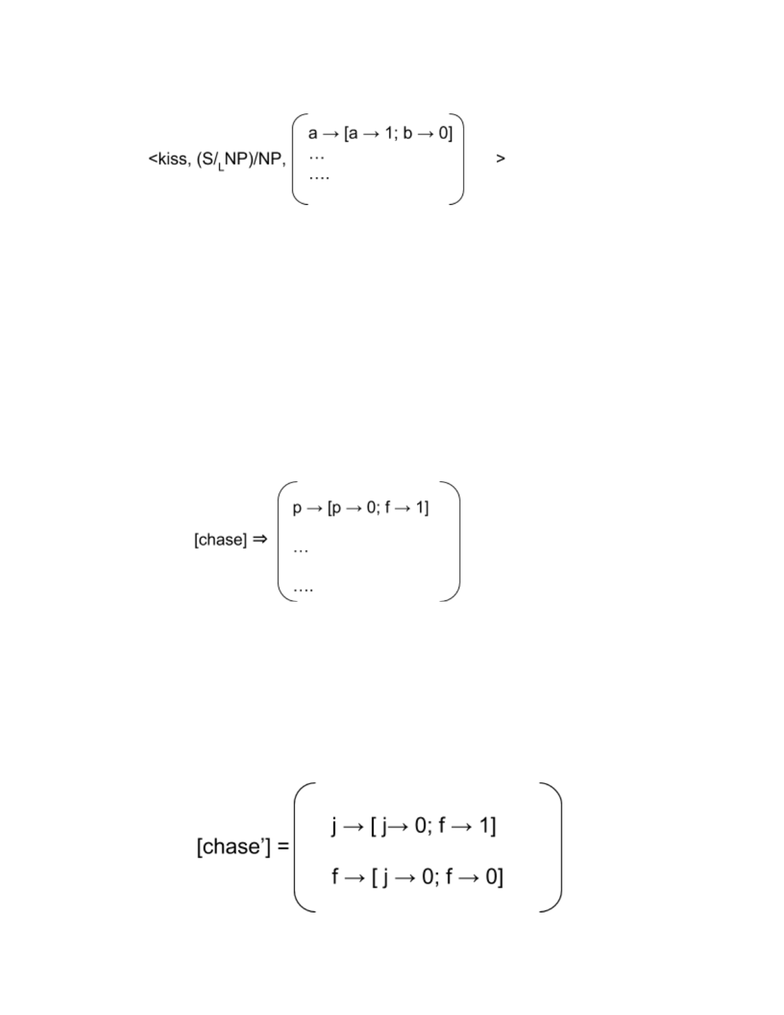Class Notes (1,100,000)
US (490,000)
UCLA (10,000)
LING (100)
Lecture 3

# LING 165C Lecture Notes - Lecture 3: Arity, Compound Verb, Formal Language

Department
Linguistics
Course Code
LING 165C
Professor
Dylan Bumford
Lecture
3

This preview shows half of the first page. to view the full 3 pages of the document.1/23/19 Lecture 4
- Notation too complicated
- Meta Language
- Semantics of the formal language
- For every formula, which object in the model does it refer to
- Language consists of
- Simple predicates: all expelled as verbs for convenience (i.e chase’, see’,
...) and each has an associated number specifying number of arguments
called arity
- Terms: will have
- Constants: names like “john”, “pinky”, “fido”
- Formula: a predicate with arity 0
- For any predicate P with arity n, and any term 𝛕: P(𝛕) is a complex predicate of
arity n - 1. (i.e chase’ has arity 2, and chase’(john’) has arity 1, and
chase’(john’)(fido’) has arity 0, which is now called a formula)
- If P has arit n, then [P] is an in-place function into truth values
- If 𝛕 is a constant, then [𝛕] is an entity in the model
- [ p(𝛕) ] = [p]( [𝛕] )
- Example of this meta language
John chases Fido.
We have only two things in the model: John and Fido
Chase is a predicate that takes in two arguments, it has the following denotation.
We read from inside out. Constituent always combine with the object first. So in the
below case, Fido chased John
[chase’ (john’)(fido’)] [ chase’(john’) ]([fido’]) = [ [chase’] ([ john’ ]) ] (‘[fido’])